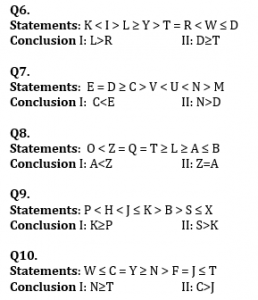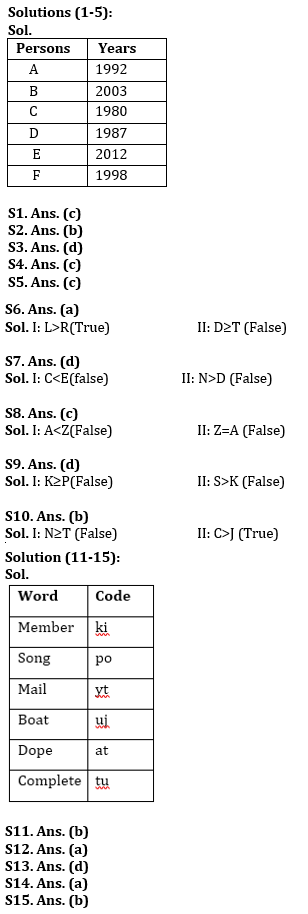Latest Banking jobs   »

# Reasoning Ability Quiz For IBPS Clerk Prelims 2022- 6th August

Directions (1-5): Study the following information carefully and answer the question given below:
Six persons were born on the same day of the same month of different year. All their ages are to be considered from the base year i.e., 2020. C is the oldest person and was born in 1980. D is 7 years younger than C and 5 years older than A. E is the youngest person in the group. B is 17 years old. Age difference between A and F is 6 years. B is younger than F who is younger than D. Age difference between A and E is 9 years more than the age difference between D and F.

Q1. In which of the following year D was born?
(a) 1992
(b) 2003
(c) 1987
(d) 2012
(e) None of these

Q2. Who among the following person is youngest person?
(a) A
(b) E
(c) B
(d) F
(e) None of these

Q3. What is the age difference between F and C?
(a) 10 years
(b) 21 years
(c) 20 years
(d) 18 years
(e) None of these

Q4. Who among the following is 22 years old?
(a) D
(b) E
(c) F
(d) A
(e) None of these

Q5. Who among the following person was born in 1992?
(a) B
(b) D
(c) A
(d) F
(e) None of these

Direction (6-10): In these questions, relationship between different elements is show in the statements. The statements are followed by conclusions. Study the conclusions based on the given statements and select the appropriate answer:
(a) If only conclusion I follows.
(b) If only conclusion II follows.
(c) If either conclusion I or II follows
(d) If neither conclusion I nor II follows.
(e) If both conclusions I and II follow.Directions (11-15): Study the following information carefully and answer the questions given below:
In a certain code language:
‘Member song mail’ is written as ‘yt po ki’,
‘Boat complete dope’ is written as ‘tu at uj’,
‘Mail dope Boat’ is written as ‘at yt uj’
‘Member mail Boat’ is written as ‘yt uj ki’.

Q11. What is the code for ‘song member’ in the given code language?
(a) tu uj
(b) po ki
(c) at uj
(d) uj po
(e) None of these

Q12. What is the code for ‘complete’ in the given code language?
(a) tu
(b) ki
(c) at
(d) uj
(e) None of these

Q13. What is the code for ‘Boat’ in the given code language?
(a) tu
(b) po
(c) at
(d) uj
(e) None of these

Q14. What may be the code for ‘mail delivered’?
(a) yt sa
(b) po ki
(c) at sa
(d) sa tu
(e) None of these

Q15. What is the code for ‘mail dope’ in the given code language?
(a) yt fa
(b) yt at
(c) uj ki
(d) yt uj
(e) None of these

Solutions#### Congratulations!Union Budget 2023-24: Free PDF